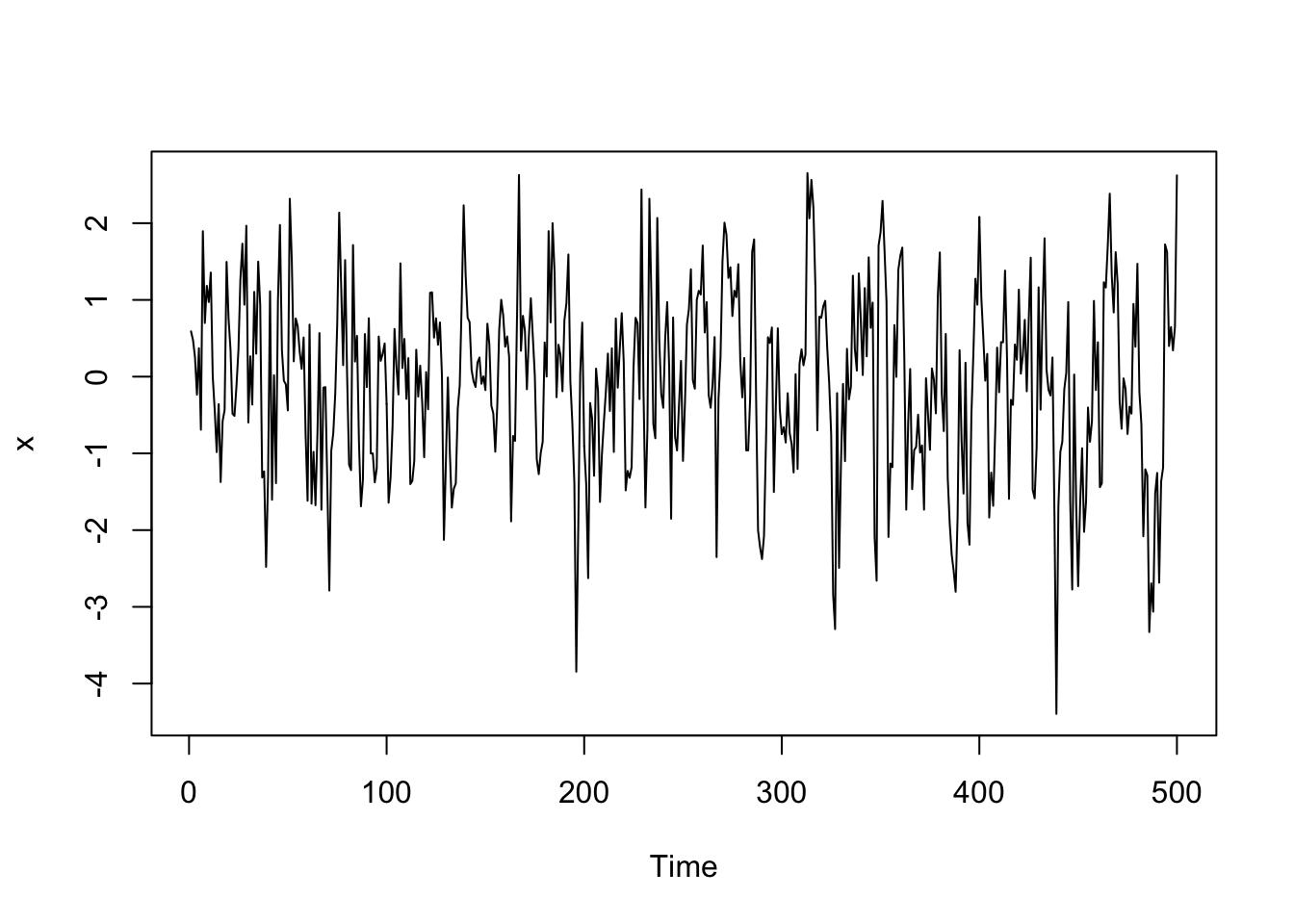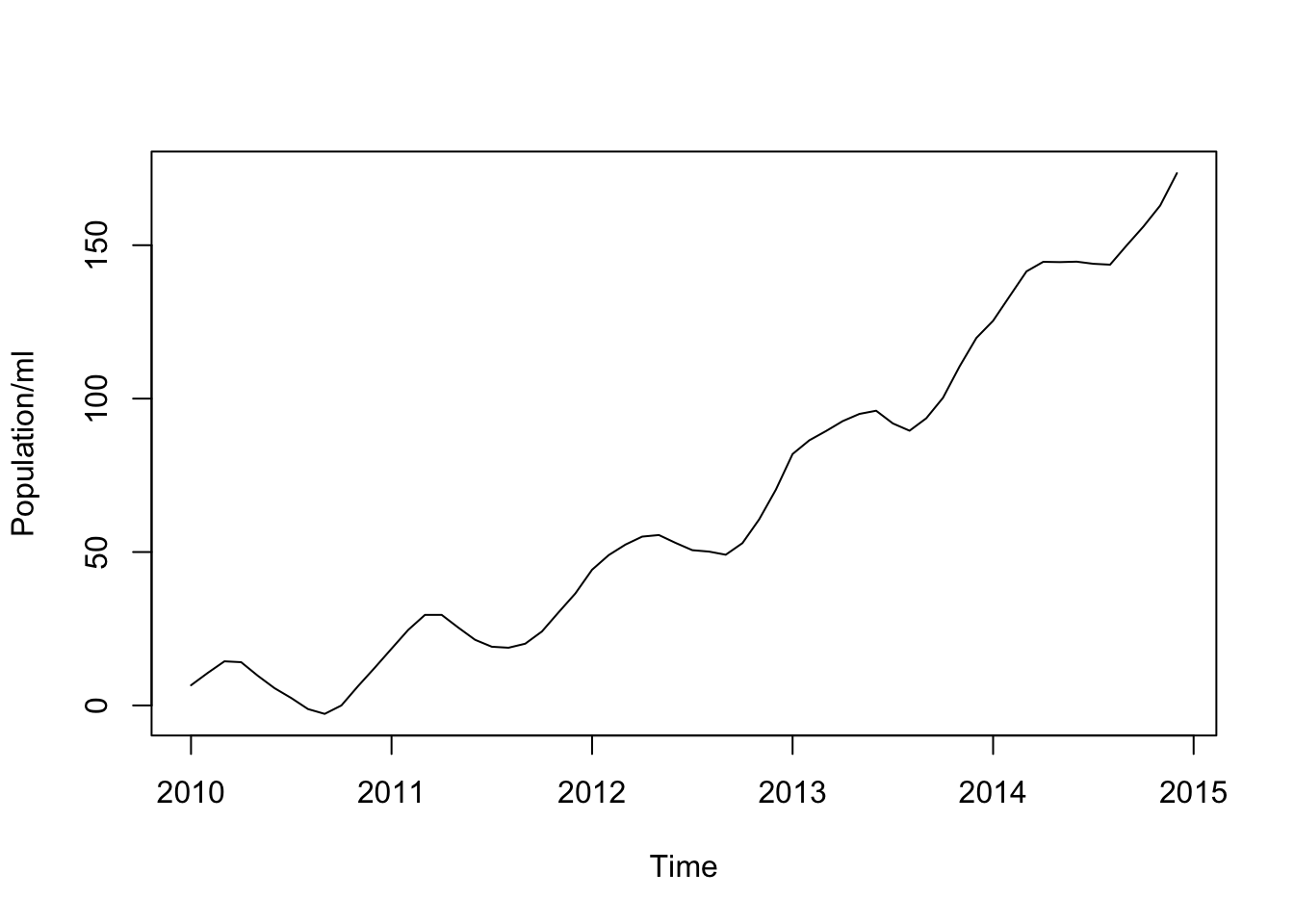## Concepts and Theoretical Questions

### Problem 1.)

#### Problem 1 Part a.): Investigate the stationarity of $$X_t$$

We will calculate the $$E[X_t]$$.

\begin{align} E[X_t] &= E[\beta_0 + \beta_1t + \beta_2\sin(\frac{\pi}{2}t) + Z_t]\\ &=\beta_0 + \beta_1t + \beta_2\sin(\frac{\pi}{2}t) + 0 \end{align}

This is not stationary since the expected value of $$X_t$$ is a function of time $$t$$.

#### Problem 1 Part b.) Show delta_4 X_t does not have a time-dependent mean

\begin{align} E[\Delta_4X_t] &= E[X_t - X_{t-4}]\\ &= E[\beta_0 + \beta_1t + \beta_2\sin(\frac{\pi}{2}t) + Z_t] - E[\beta_0 + \beta_1(t-4) + \beta_2\sin(\frac{\pi}{2}(t-4)) + Z_{t-4}]\\ &=\beta_0 + \beta_1t + \beta_2\sin(\frac{\pi}{2}t) + 0 - \beta_0 - \beta_1t + 4\beta_1 - \beta_2\sin(\frac{\pi}{2}(t-4))+ 0\\ &=4\beta_1 \end{align}

Note that $$\beta_2\sin(\frac{\pi}{2}t)$$ and $$\beta_2\sin(\frac{\pi}{2}(t-4))$$ are equal because they are sinuisoidal function with period 4. Thus, in the above derivation they cancel each other out.

We conclude that $$E[\Delta_4X_t] = 4\beta_1$$, which is independent of time $$t$$.

#### Problem 1 Part c.): Compare the two differencing procedures

We will compare the variance of each differencing procedure.

\begin{align} Var(\Delta_4X_t) &= E[(\Delta_4X_t)(\Delta_4X_t)] - (E[\Delta_4X_t])^2\\ &= E[(X_t - X_{t-4})^2] - (E[\Delta_4X_t])^2\\ &=E[X_t^2 - 2X_tX_{t-4} + X_{t-4}^2]\\ &= ...\\ &= 2\sigma^2_z + 16\beta_1^2 - (4\beta_1)^2\\ &=2\sigma^2_z \end{align}

The algebra involved in this derivation is neither interesting nor informative. Some things that helped that are worth noting are: $$\sin(\frac{\pi}{2}t)$$ is periodic 4, $$Z_t$$ and $$Z_{t-4}$$ are independent and so the product of their expectations factor, and that since $$Z_t \sim WN(0,\sigma^2_z)$$, it follows that the second moment of $$Z_t$$ is equal to its variance $$\sigma^2_z$$

We now calculate the Variance of the alternative differencing procedure.

\begin{align} Var[\Delta_4\Delta X_t] &= Var[\Delta_4(X_t - X_{t-1})] \\ &=E[(\Delta_4X_t - \Delta_4X_{t-1})(\Delta_4X_t - \Delta_4X_{t-1})] - (E[\Delta_4X_t -\Delta_4X_{t-1}])^2\\ &=E[(\Delta_4X_t)(\Delta_4X_t)] + E[(\Delta_4X_{t-1})(\Delta_4X_{t-1})] - 2E[(\Delta_4X_t)(\Delta_4X_{t-1})] - (E[\Delta_4X_t -\Delta_4X_{t-1}])^2\\ \end{align}

We will calculate this Variance term by term (there are 4 terms). We will show that the first two terms are equal, and that they both equal $$2\sigma^2_z + 16\beta_1^2$$. We will then show that the fourth term is equal to 0. Then we will show the third term is equal to $$16\sigma^2_z$$

Now, we already know the first term $$E[(\Delta_4X_t)(\Delta_4X_t)] = 4\beta_1$$ (by Problem 1 Part b.), and furthermore we already calculated $$Var(\Delta_4X_t) = 2\sigma^2_z$$ earlier in this part c. Thus, we have:

$E[(\Delta_4X_t)(\Delta_4X_t)] = 2\sigma^2_z + 16\beta_1^2$ Similarly, since neither of these values depended on timee $$t$$, we can also conclude that the second term is equal. In other words we have:

$E[(\Delta_4X_{t-1})(\Delta_4X_{t-1})] = 2\sigma^2_z + 16\beta_1^2$

The third term. The algebra is neither interesting nor informative, so details will be omitted.

$2E[(\Delta_4X_t)(\Delta_4X_{t-1})] = 2(16\beta_1^2)$

Finally the fourth term. We have:

\begin{align} (E[\Delta_4X_t -\Delta_4X_{t-1}])^2 &= (E[\Delta_4X_t] - E[\Delta_4X_{t-1}])^2\\ &= (4\beta_1 - 4\beta_1)^2\\ &= 0\\ \end{align}

Therefore, we have the following:

\begin{align} Var[\Delta_4\Delta X_t] &= Var[\Delta_4(X_t - X_{t-1})] \\ &=E[(\Delta_4X_t - \Delta_4X_{t-1})(\Delta_4X_t - \Delta_4X_{t-1})] - (E[\Delta_4X_t -\Delta_4X_{t-1}])^2\\ &=E[(\Delta_4X_t)(\Delta_4X_t)] + E[(\Delta_4X_{t-1})(\Delta_4X_{t-1})] - 2E[(\Delta_4X_t)(\Delta_4X_{t-1})] - (E[\Delta_4X_t -\Delta_4X_{t-1}])^2\\ &= 2\sigma^2_z + 16\beta_1^2 + 2\sigma^2_z + 16\beta_1^2 - 2(16\beta_1^2) + 0\\ &= 4\sigma^2 \end{align}

In conclusion we have:

$Var[\Delta_4X_t] = 2\sigma^2\\ Var[\Delta_4\Delta X_t] = 4\sigma^2$

Since $$\Delta_4X_t$$ has smaller variance, we would choose $$\Delta_4X_t$$ as our differencing procedure.

### Problem 2

#### Find the mle of phi

We assume $$x_1$$ is known. We calculate the conditional likelihood of $$\theta = (\phi, \sigma^2_w)^T$$ given $$x_1$$.

\begin{align} L(\theta) &= f_\theta(x_2,...,x_n|x_1)\\ &=\frac{f_\theta(x_2,...,x_n)}{f_\theta(x_1)}\\ &=\frac{f_\theta(x_n|x_{n-1},...,x_1)*f_\theta(x_{n-1}|x_{n-2},...,x_1)*...*f_\theta(x_2|x_1)*f_\theta(x_1)}{f_\theta(x_1)}\\ &=f_\theta(x_n|x_{n-1},...,x_1)*f_\theta(x_{n-1}|x_{n-2},...,x_1)*...*f_\theta(x_2|x_1)\\ &=\prod_{t=2}^n f_\theta(x_t|x_{t-1}) \end{align}

Where the last line of equality follows due to the 1-dependency of the AR(1) model. In other words, $$X_t$$ depends only on the previous value $$X_{t-1}$$

Now we need to analyze the conditional distribution of each $$X_t|X_{t-1}$$. We first note that $$X_t|X_{t-1}$$ is Normally distributed. Thus we seek its Expected Value and Variance. Once we have those we can write the conditional density.

$E[X_t] = \phi X_{t-1}\\ Var[X_t] = \sigma^2_w\\ f_\theta(x_t|x_{t-1}) = \frac{1}{\sqrt{2\pi\sigma^2_w}}\exp(-\frac{(x_t-\phi x_{t-1})^2}{2\sigma^2_w})$

We used basic linearity properties of $$E[]$$ a $$Var[]$$ to calculate the Expected Value and Variance.

To find the mle of $$\phi$$ we take the log of the likelihood and maximize with respect to $$\phi$$.

$\ell(\theta) = \sum_{t=2}^n \log(f_\theta({x_t|x_{t-1}}))\\ \hat{\phi} = argmax_\phi \ell(\theta)$

We take the partial derivative with respect to $$\phi$$ and set equal to zero.

$\frac{\partial}{\partial\phi} = -\frac{1}{2\sigma^2_w}\sum_{t=2}^n -2(x_t - \phi x_{t-1})(x_{t-1}) = 0$

Solving this equation for $$\phi$$ gives

$\hat{\phi}_{MLE} = \frac{\sum_{t=2}^n x_t x_{t-1}}{\sum_{t=2}^n x_{t-1}^2}$

### Problem 3.)

Multiple Choice B

### Problem 4.)

Multiple choice B and C

### Problem 5.)

Multiple choice C

### Problem 6.)

Multiple choice B and C are TRUE.

### Problem 7.)

Multiple choice A and F are non-stationary.

## Simulation/Data Analysis

### Problem 8.)

set.seed(100)
x <- arima.sim(model=list(order=c(1,0,0), ar=0.6), n=500)
plot.ts(x)fit <- arima(x, order=c(1,0,0))
fit
##
## Call:
## arima(x = x, order = c(1, 0, 0))
##
## Coefficients:
##          ar1  intercept
##       0.5148    -0.0847
## s.e.  0.0385     0.0933
##
## sigma^2 estimated as 1.028:  log likelihood = -716.61,  aic = 1439.23
#MLE calculation using formula
numerator = 0
denominator = 0
for(i in 2:500) {
x.t = x[i]
x.t1 = x[i-1]
numerator = numerator + (x.t*x.t1)
denominator = denominator + x.t1^2
}
phi.hat = numerator/denominator
print(paste('phi.hat = ', phi.hat))
##  "phi.hat =  0.518499668215625"

Using the formula I derived in Problem 2 I found that $$\hat{\phi} \approx 0.5184$$

Using the R’s arima() function the estimate of $$\hat{\phi}$$ is 0.5148. These two estimates are fairly close (off by 1000th decimal place).

### Problem 9.) Population.csv

#### Part a.)

data = read.csv('Population.csv')
myts <- ts(data[,2], start=c(2010, 1),frequency=12)
plot.ts(myts, ylab='Population/ml')There appears to be a general increasing trend. There also appears to be a seasonal trend. In particular, the last few months of every year going into the first few months of every year, i.e. the Winter and early Spring months, tend to show an increase in the population of count per milliliters of rotifers. I do not notice any anomalies.

#### Part b.): Decomposition of TS

population_dcomp = decompose(myts, 'additive')
plot(population_dcomp, yax.flip=TRUE)